#### How to do a Multiple Regression in Excel

In this Excel tutorial, you will learn how to run a multiple regression and how to interpret the results.

The multiple regression is the way how to check the relationship of two features.

In order for the model to be as reliable as possible, the largest possible number of independent variables should be introduced into the model. The model should contain variables strongly correlated with the dependent variable and at the same time as weakly correlated with each other as possible.

## Examples of multiple regression

• The volume of sales depends on the proportion in which the advertising campaign was carried out in three different local newspapers. Question: What is the proportion of spending on an advertising campaign in these newspapers so that the sale is the highest?
• The appraisal of an apartment depends on many characteristics of the property, such as: area, number of rooms, age, type of heating, district, number of floors. From the developer’s point of view, the analysis will show the value of the apartment he is going to build. Maybe it is worth adding a garage?
• The bank wants to check whether it should grant the customer a loan. He knows his income, education, age, savings, marital status, number of children. Based on the analysis of these features, the bank wants to receive data on the risk that the customer will not repay the loan. If a high risk of loan default is identified, the bank will refuse to grant a loan based on a regression analysis.
• A good example is also the study of life expectancy based on factors such as: neighborhood, health condition, medications taken, physical activity, etc.

## How to calculate?

The multiple regression in Microsoft Excel is possible. The best method is a step-by-step instruction that will show you how. The first thing is having a data that could look something like this: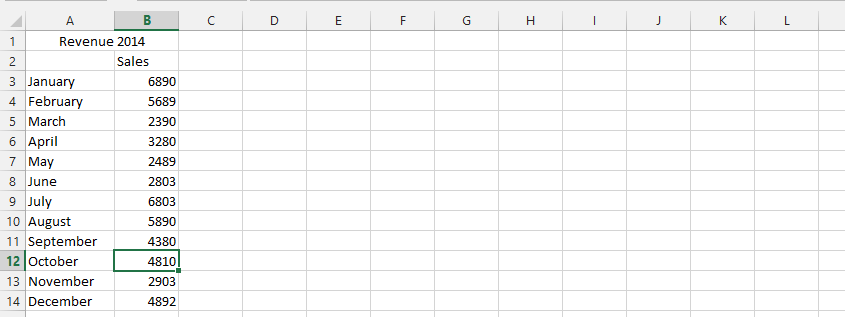1. Click on data (1), and then click on the data analysis (2)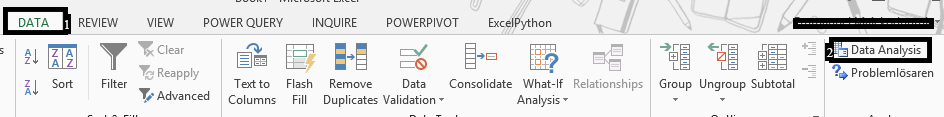Note: The data analysis could be gotten from the add-in (file > options > add-ins > Go).

2. Choose Regression in the Data Analysis, and click ok.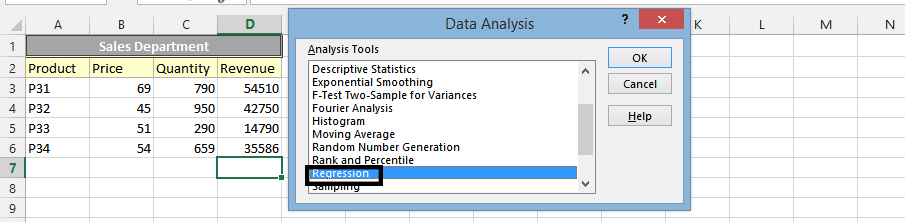3. Choose the data you’d want to analyze on X values (1), and Y values (2). Select desired options, under the residuals (3), and press ok (4).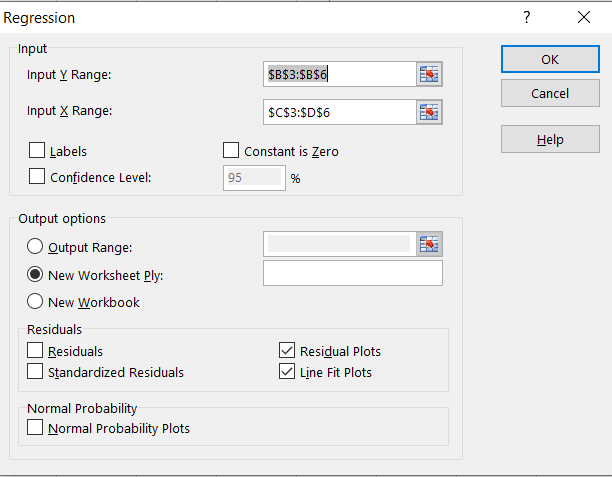In conclusion, the multiple regression would look something like this: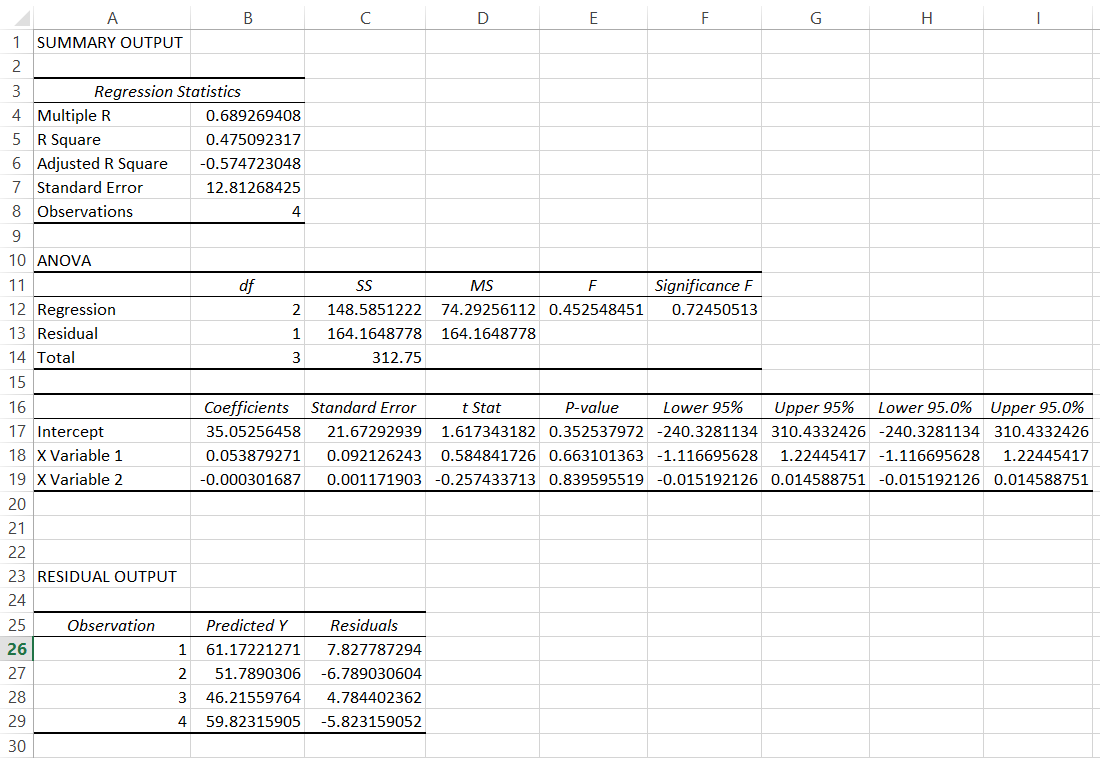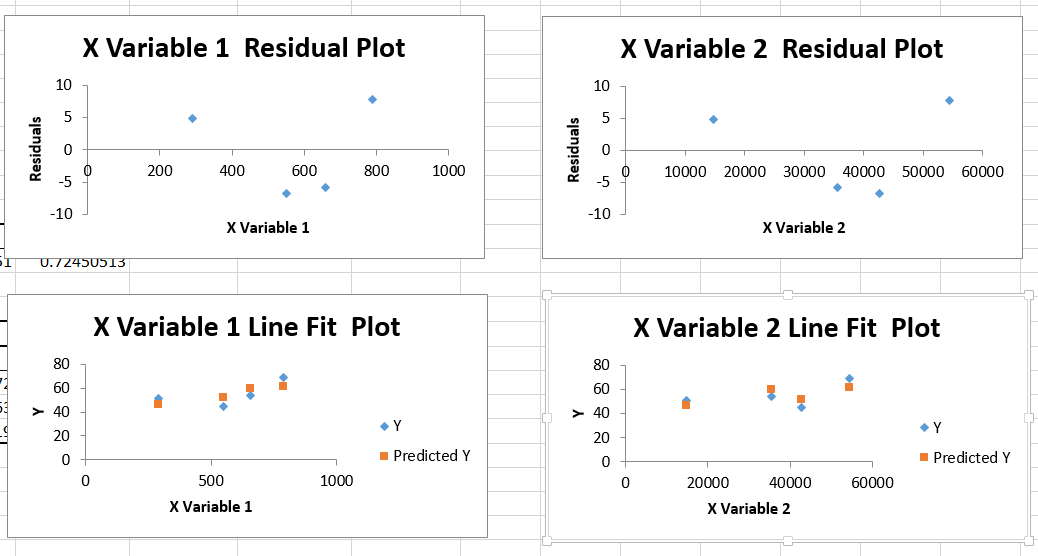## Interpreting the results

### Regression Statistics

• Multiple R (aka. Correlation Coefficient) is higher than 0. It means that there is a positive relationship. The higher the number (the closer to 1) the stronger the relationship.
• R square (aka. Coefficient of Determination) is equal to 0.47. That means 47% of values fit to the model of our analysis.
• Adjusted R Square you should focus on that in the situation of having more than one x values.
• Standard error is very high. This is the information that I cannot be confident about the analysis too much because of the high error I need to remember about.
• Observations is not what need to be analyzed. This is the number of observations provided by me to the analysis.

### Anova

• F is a F-test of the null hypothesis and the Significance F is a p-value of F.
• Significance F is the most important here. The first thing to note is that this number is much higher than alpha (0.5), meaning the observation is not statistically significant.